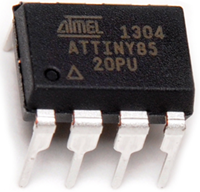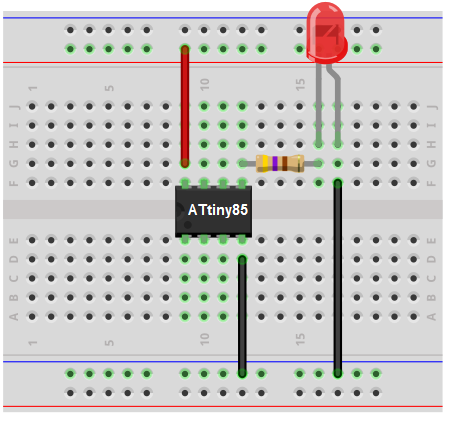﻿ How to Build an LED Blinker Circuit with an ATtiny85

# How to Build an LED Circuit with an ATtiny85 MicrocontrollerIn this project, we will build an LED circuit with an ATtiny85 microcontroller.

This circuit will blink an LED on and off, on and off, indefinitely.

This project serves as an introduction of how to turn on and off a digital output device, in this case an LED.

Once you know how this circuit works, you will know how to turn on or off any digital device using an ATtiny85 chip. So it's a powerful introductory circuit.

The ATtiny85 microcontroller has 6 I/O pins. This means we can connect up to 6 output devices to the microcontroller. However, we will only connect 1 LED in this circuit and show how ot turn it on and turn it off.

Blinking a digital device such as an LED is achieved primarily through code. We will show in our code how this is accomplished.

### Components Needed

• ATtiny85 microcontroller
• LED
• 470Ω Resistor

The ATtiny85 microcontroller can be obtained through many electronic online retailers. One easy place to purchase it is ebay. Depending on where you get and the quantity you get, it can range anywhere from a little over \$1-\$4.

The datasheet for the ATtiny85 microcontroller is shown at the following link: ATtiny85 Datasheet.

The ATtiny85 chip is an 8-pin microcontroller.

The pinout of the ATtiny85 is shown below.The power pins are VCC and GND, pins 8 and 4. Anywhere from 1.8V to 5.5V can power the ATtiny85 chip. To our circuit, we will simply apply 5V to VCC and connect GND to power ground.

Besides the 2 power pins discussed right above, the other 6 pins of the ATtiny8 are I/O pins labeled as PORTB pins. These are labeled on the datasheet and in the pinout above as PB0 to PB5.

These pins can be used as inputs or outputs, and they have various uses depending on what you need the pin for.

In our circuit, we are simply using the I/O pins or PORTB pins as outputs.

The PORTB pins of the ATtiny85 source power, so when connecting a digital output, the port provides positive power to the output device, so that we just need to connect the other end of the device to ground.

The circuit diagram of the blinking LED circuit we will building with an ATtiny85 microcontroller is shown below.The breadboard schematic of the circuit above is shown below.First, we give power to the ATtiny85 chip. This is done by connecting 5V to VCC, pin 8, and by connecting GND, pin 4, to ground.

Next, we attach to the output of the ATtiny85 microcontroller an LED. This LED will be attached to the pin PB0.

There are 3 more outputs avaiable on the ATtiny85, PB3, PB4, and PB5. However, we are only using the first 3 outputs, but you can easily extend it to all 6 outputs.

Being that the outputs source current, we connect the anode terminal of the LED directly to the output and the cathode end gets connected to ground. This supplies sufficient power to the LEDs. Being that LEDs only require about 10mA-20mA of current, they don't use that much power. However, for devices that need significantly more current such as a motor, for instance, we would have to connect a transistor to the output and then attach the output device to the collector of the transistor. This gives current amplification so that it can drive high-current devices. But, again, for LEDs, this is not necessary.

This establishes all the connections that are needed for this circuit.

Now all we need is the code.

### Code

The code needed in order to turn all the LEDs on is shown below.

#include <avr/io.h>
#include <util/delay.h>

int main(void)
{
while(1)
{
DDRB= 0xff; //sets all the pins as outputs
PORTB= 0xff; //set all of PORT B pins as HIGH
_delay_ms(500);//keeps the LED on for a half a second
PORTB= 0x00;//turns the LED off
_delay_ms(500);//keeps the LED off for a half a second
}
}

First, we must important the <avr/io.h> library.

Then we create the main loop() which will house the while(1) loop. This loop is an infinite loop. So the program will keep executing the code contained inside of it infinitely, over and over again. In this loop, we declare the DDRB pin as 0xff, which makes all of the I/O pins of the ATtiny85 outputs.

In the next line, PORTB= 0xff;, we set all the outputs HIGH, which turns the outputs on. Therefore, the LED at pin PB0 turns on. We call a delay of 500 which creates a delay of a half a second.

The _delay_ms() function creates a delay. The number that you place inside the parameter creates that numerical value in delay in milliseconds. A parameter of 500 creates a delay of 500 milliseconds, which is a half a second. You can modify this value to any number. If you want the LED to be on for a full second, the number in the parameter would be 1000. If you want the LED to be on for 3 seconds, the number is 3000. Since there are 1000 milliseconds in one second, if you know the amount of seconds you want the delay in, you simply multiply this number by 1000 to get the numerical value you want place in the _delay_ms() function. Since we are creating a delay of a half a second, or 0.5 seconds, 0.5 * 1000= 500, which is what we use in this program.

In the next line, PORTB= 0x00;, we turn all of the outputs off, so the LED turns off. We then call another delay of 500 milliseconds, a half a second. So the LED turns off for a half a second.

The loop then starts again, which turns the LED on for a half a second, then off for a half a second, and continues over and over and over forever, unless power is shut off to the circuit.

And this is how we can turn a digital device on and off, or blink the device, at any pace we want to.

And this is a simple program to control output digital devices.

Related Resources